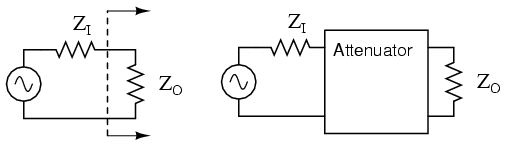# attenuator

Attenuators are passive devices. It is convenient to discuss them along with decibels. Attenuators weaken or attenuate the high level output of a signal generator, for example, to provide a lower level signal for something like the antenna input of a sensitive radio receiver. The attenuator could be built into the signal generator, or be a stand-alone device. It could provide a fixed or adjustable amount of attenuation. An attenuator section can also provide isolation between a source and a troublesome load.Constant impedance attenuator is matched to source impedance ZI and load impedance ZO. For radio frequency equipment Z is 50 Ω.

## RF/microwave attenuator

In many microwave systems attenuators are required for automatic gain control of RF receiver and transmitter systems. Fixed and variable microwave attenuators provide gain level adjustments for microwave circuits. Attenuators are also used for amplitude weighting in phased array radar and for temperature compensation of microwave amplifiers. Generally, microwave attenuators operate by absorbing the electromagnetic energy of a wave traveling down the wave guide. The amount of attenuation may be varied by changing the insertion depth of an absorbing element.

In the case of a stand-alone attenuator, it must be placed in series between the signal source and the load by breaking open the signal path as shown above. In addition, it must match both the source impedance ZI and the load impedance ZO, while providing a specified amount of attenuation. In this section we will only consider the special, and most common, case where the source and load impedances are equal. Not considered in this section, unequal source and load impedances may be matched by an attenuator section. However, the formulation is more complex.T section and Π section attenuators are common forms.

Common configurations are the T and Π networks shown in Figure above
Multiple attenuator sections may be cascaded when even weaker signals are needed as in Figure

### Decibels

Voltage ratios, as used in the design of attenuators are often expressed in terms of decibels. The voltage ratio (K below) must be derived from the attenuation in decibels. Power ratios expressed as decibels are additive. For example, a 10 dB attenuator followed by a 6 dB attenuator provides 16dB of attenuation overall.

10 dB + 6 db = 16 dB

Changing sound levels are perceptible roughly proportional to the logarithm of the power ratio (PI / PO).

sound level = log10(PI / PO)

A change of 1 dB in sound level is barely perceptible to a listener, while 2 db is readily perceptible. An attenuation of 3 dB corresponds to cutting power in half, while a gain of 3 db corresponds to a doubling of the power level. A gain of -3 dB is the same as an attenuation of +3 dB, corresponding to half the original power level.

The power change in decibels in terms of power ratio is:

dB = 10 log10(PI / PO)

Assuming that the load RI at PI is the same as the load resistor RO at PO (RI = RO), the decibels may be derived from the voltage ratio (VI / VO) or current ratio (II / IO):

PO = V O IO = VO2 / R = IO2 R

PI = V I II = VI2 / R = II2 R

dB = 10 log10(PI / PO) = 10 log10(VI2 / VO2) = 20 log10(VI/VO)

dB = 10 log10(PI / PO) = 10 log10(II2 / IO2) = 20 log10(II/IO)

The two most often used forms of the decibel equation are:

dB = 10 log10(PI / PO)      or         dB = 20 log10(VI / VO)

We will use the latter form, since we need the voltage ratio. Once again, the voltage ratio form of equation is only applicable where the two corresponding resistors are equal. That is, the source and load resistance need to be equal.

Example:

Power into an attenuator is 10 watts, the power out is 1 watt. Find the attenuation in dB.

dB = 10 log10(PI / PO) = 10 log10 (10 /1) = 10 log10 (10) = 10 (1) = 10 dB

Example:

Find the voltage attenuation ratio (K= (VI / VO)) for a 10 dB attenuator.

dB = 10= 20 log10(VI / VO)

10/20 = log10(VI / VO)

1010/20 = 10log10(VI / VO)

3.16 = (VI / VO) = AP(ratio)

Example:

Power into an attenuator is 100 milliwatts, the power out is 1 milliwatt. Find the attenuation in dB.

dB = 10 log10(PI / PO) = 10 log10 (100 /1) = 10 log10 (100) = 10 (2) = 20 dB

Example:

Find the voltage attenuation ratio (K= (VI / VO)) for a 20 dB attenuator.

dB = 20= 20 log10(VI / VO )

1020/20 = 10 log10(VI / VO )

10 = (VI / VO ) = K

More details ./link/attenuators.html

on line design software for attenuator , free

An Offset Attenuator for Radio Direction Finding

Attenuator Design Tutorial

Attenuators application notes

Attenuators, with use of PIN diode

variable Attenuators application notes

Bridged T Pad Attenuator Design articles

design of Offset Attenuator

Evaluating Attenuators article ; Reliability Resistance

Design Software includes stepped attenuator

Designing a symmetric matched resistive T-attenuator ;Homework

Minimum Loss Attenuator Design Guide

Design Tee Attenuator To Minimize Stress article

Resistive Attenuator Design

Attenuator Dissipation
Equations for calculating the fractional dissipation of the individual resistors in an attenuator.

design of attenuator with calculation the resistor values

Related

COMMENT Uncategorized

EDUCATION

INSURANCE

CONTACT
FAVORITE
SITEMAP
VLSI COMPANIES IN BANGALORE
VLSI INTERVIEW QUESTION RFSIR.COM